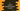# C program to convert a decimal number to octal### Convert a decimal number to octal in C :

In this tutorial, we will learn how to convert a decimal number to octal in c programming language. The octal number system is a base-8 number system. To represent one number in octal, digits from 0 to 7 is used. If a number is in decimal and if we want to convert it to octal, how to achieve it? Let’s take a look at a simple example : Suppose we want to convert the number 100 to octal. We will keep dividing this number by 8 and keep appending the remainder till the number becomes 0 :

``````100 % 8 = 12 (quotient) / 4 (remainder) --> 4 (result)
12 % 8 = 1 (quotient) / 4 (remainder) --> 44 (result)
1 % 8 = 0 (quotient) / 1 (remainder) --> 144 (result)
quotient is 0 --> stop
``````

To convert a decimal number to octal programmatically using the above method, let’s take a look into the C program first :

### C program to convert a decimal number to octal :

``````#include <stdio.h>

//5
int convertToOctal(int no)
{
//6
int octal = 0;
int multiplier = 1;

//7
while (no != 0)
{
//8
octal = octal + (no % 8) * multiplier;
no = no / 8;
multiplier = multiplier * 10;
}

//9
return octal;
}

int main()
{
//1
int decimalNo;

//2
printf("Enter a Decimal Number : ");

//3
scanf("%d", &decimalNo);

//4
printf("Octal representation for %d is %d\n", decimalNo, convertToOctal(decimalNo));
}``````

### Explanation :

1. Create one integer variable decimalNo to store the user input number.
2. Ask the user to enter a number.
3. Save the number in variable decimalNo.
4. Pass the integer to function convertToOctal() to find out the octal representation of the decimal number.
5. int convertToOctal(int no) method is used to convert a decimal to octal. It takes one decimal number as input and returns the octal representation of that number.
6. Create one variable to save the octal representation and one variable multiplier.
7. Start one while loop and this loop will run till the number becomes zero 0.
8. Find out the octal representation of the number by repeating the same process as shown in the example above.
9. return the final octal representation of the number to the caller function.

### Sample Output :

``````Enter a Decimal Number : 100
Octal representation for 100 is 144

Enter a Decimal Number : 200
Octal representation for 200 is 310

Enter a Decimal Number : 300
Octal representation for 300 is 454
``````